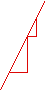# Teachers' MathematicsMath Lessons for Math Teachers
by Henri Picciotto

Participants in the debates on math education argue about many things, but they seem to agree on at least one idea: math teachers should know math, the more the better. Unfortunately, many math teachers in the United States have not studied math much beyond the classes they teach. Others have, but found that college-level mathematics courses are not all that related to the K-12 curriculum.

One solution lies in developing preservice and in-service education that helps teachers develop more depth of understanding of pre-college math. Zalman Usiskin considers this field a part of applied math, and calls it Teachers' Mathematics. Ideally, each math teacher and each math department would be engaged in continuous studying of teachers' mathematics, in a way that is intimately connected with classroom teaching. Unfortunately, given how little preparation time teachers have, much of this has to happen at conferences and during summer workshops.

On this page, I link to lessons in teachers' mathematics which I have used with middle school and high school teachers. Most of the lessons are based on ideas that are accessible to students, though not at the same depth.

The lessons fall more or less in three categories:

## Concept Exploration

Look at familiar material from unfamiliar angles, in order to increase depth of understanding.
Function diagrams
Iterating linear functions
Exponential Functions
Abstract Algebra
Taxicab Geometry

## Problem Analysis

Start with a problem that can be posed to students, and end with an analysis at a deeper level, typically by seeking generalization and/or proof.
The "Mc Nuggets Problem"
Staircases
Pattern Block Trains
Soccer Angles
Seems Isosceles!
Blog posts:
Scissors Congruence
Geobard Problems for Teachers
K-12 Unsolved

## Formal Development

Prove familiar results that are usually accepted without proof.
Proof of Pick's Formula
Geometry of y=mx+b
Geometry of the Parabola: 2D | 3D
Geometry of the Conic Sections
A New Path to the Quadratic Formula:
Worksheet
Article
Slides: Online | Keynote (3.2 MB)
(background: Constant Sums, Constant Products)

Summer Workshops for Math TeachersUniversity-level math: my MA thesis (UC Berkeley, 1971)
Topics in finite projective geometry